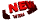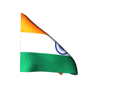Please check "WHAT IS NEW?" to see new pages we are adding. Enjoy
http://www.w2newspapers.com/ and https://newspapersallin.blogspot.in/ are same websites .use any one

Mathematics

# Mathematics

```

});

Mathematics
01.Abacus
Detailed introduction to the Abacus. History, interactive tutorial, etc.
02.AMSER
Applied math and science education repository. Links to outstanding resources.
03.Brightstorm: Math
More than 2,000 short-form online video lessons by professional math teachers.
04.Double Division
Very creative variation on long division that reduces common frustrations.
05.Euclid
Theoretical and applied mathematics and statistics journals.
06.Figure This!
Interesting math challenges for middle-school students to try at home.
07.Free Math Help
Math lessons, math games, and math help message boards.
08.Illuminations
Math resources for teachers, educators, and administrators.
09.Math Dictionary for Kids
Very creative and concise guide to hundreds of math concepts.
10.Math Planet
High school math courses in Pre-algebra, Algebra 1, Algebra 2 and Geometry.
11.Math TV
Professional video lessons in mathematics. Covers basic math through calculus.
12.Mathworld
Authoritative resource for math definitions and explanations of concepts.
13.Stack Exchange Mathematics
Online math question and answer site with an active community.
14.That Quiz
Hundreds of free online quizzes to test your math knowledge.
15.Plus Magazine
Articles on mathematics and its applications to science, art and society.
16.The Math Forum
Mathematics library and learning center by Drexel University.
17.Triple A Math
Interactive math lessons on subjects ranging from addition to exponents.
18.WebMath
Generates easy-to-understand explanations to math problems in real-time.
19.Cool Math
Math games, puzzles, and lessons.
20.Math is Fun
Easy-to-understand math puzzles, games, and worksheets.
21.Math Playground
Interactive math lessons on subjects ranging from addition to exponents.

```